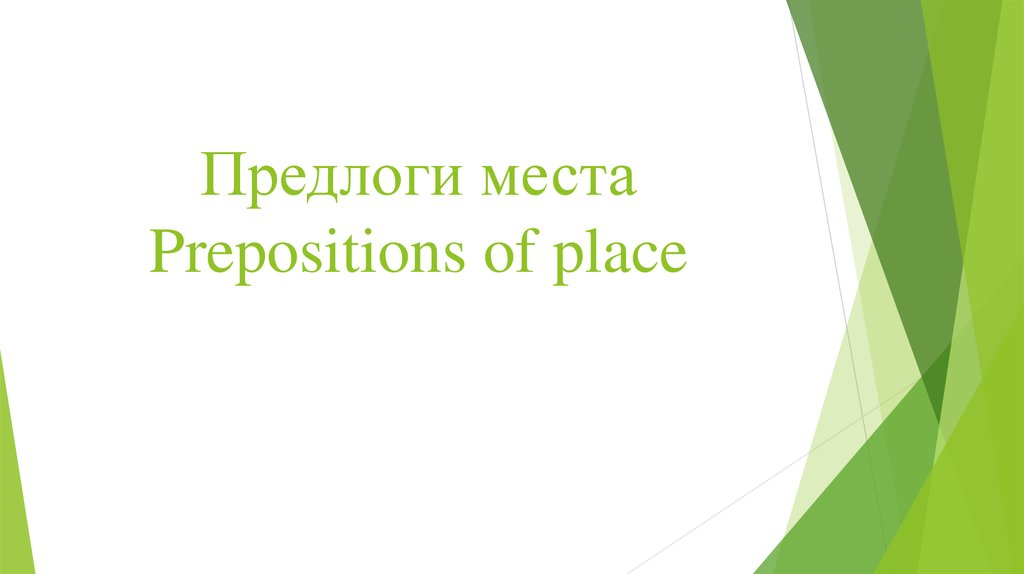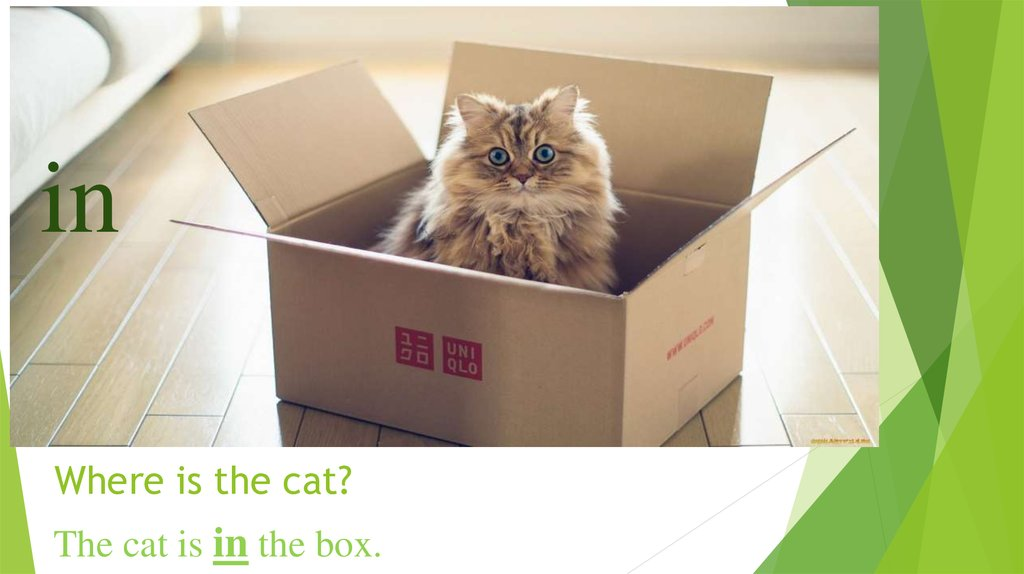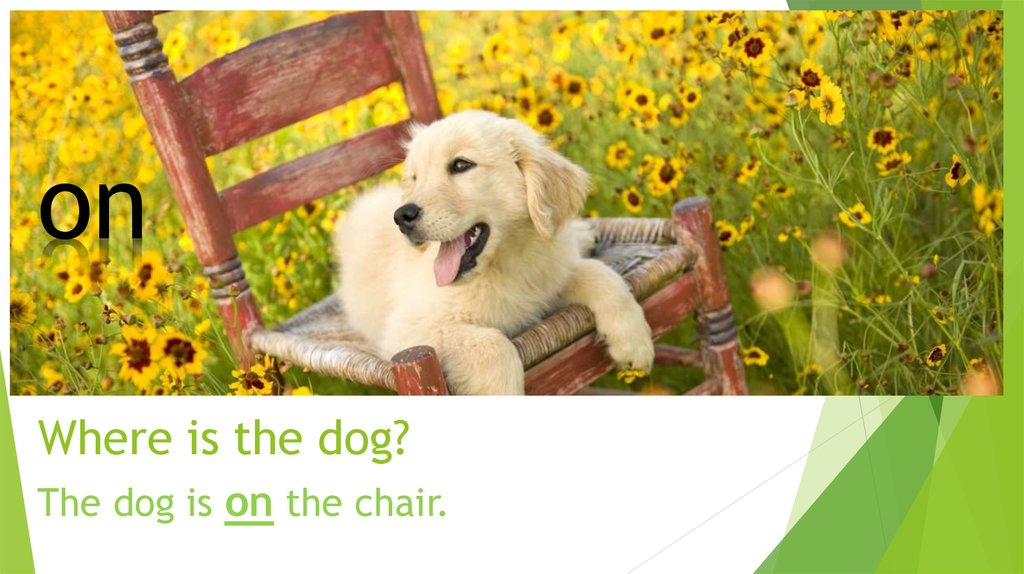# Prepositions of place

## 2. Where is the cat?

in
Where is the cat?
The cat is in the box.

## 3. Where is the dog?

on
Where is the dog?
The dog is on the chair.

## 4. Where is the bear?

The bear is under the water.

## 5. Where is the rabbit?

The rabbit is behind
the box.

## 6. Where is the cat?

The cat is next to the fox.
Where is the fox?
The fox is next to the
cat.

## 7. Where is the dog?

The dog is in front of the camera.